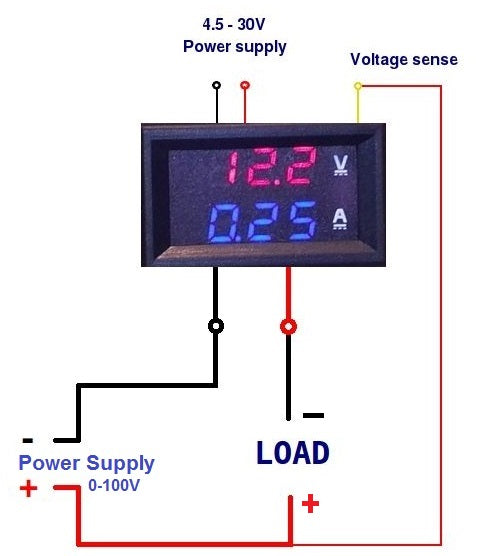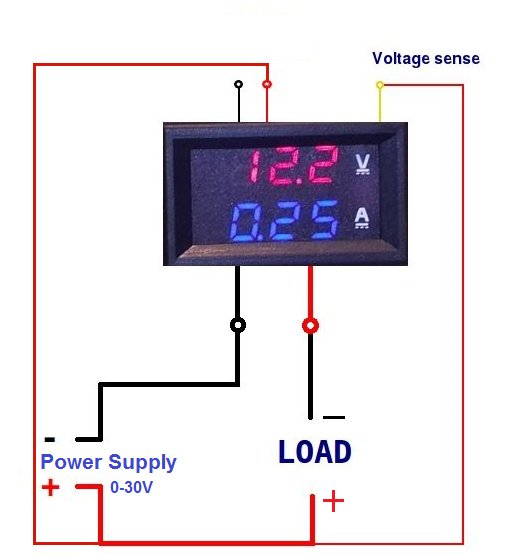The device is capable of performing two functions at once, that is it is capable of detecting both voltage and current simultaneously. The voltage is displayed at the top in red, while the current is displayed in blue at the bottom. The device has a voltage supply range of 4-30V, it can measure voltage ranging from 0-100V and current ranging from 0-10A. The device has 5 wires divided in to two sections of 3 and 2 wires.

We can use the device in two configurations, one where we supply power to the device using an external power source and one where the device draws power from the supply directly.

## (i) 1st Configuration

• In this configuration we are going to supply power to our device through a separate source.
• Connect the thin red and black wire of the meter to the separate power source used specifically to power our meter (ensure that the separate power supply is somewhere between 4-30V).
• Connect the thin yellow wire of the meter to the positive terminal of the power supply which we will use to power up the load.
• Connect the thick black wire of the meter to the power supply which we will be using to power our load.
• Connect the thick red wire of the meter to one end of the load.
• Connect the positive terminal of the supply which we will use to power the load to the free terminal of the load.
Connect the meter according to the given instructions or follow the schematic given below to easily understand the configuration.## (ii) 2nd Configuration

• In this configuration the meter is using the same power supply as the load.
• Connect the thin red and yellow wire of the meter to the positive terminal of the supply.
• Leave the thin black wire of the meter un-connected.
• Connect the positive terminal of the wire to one end of the load.
• Connect the negative terminal of the power supply to the thick black wire of the meter.
• Connect the free end of the load to the thick red wire of the meter.
Connect the meter according to the above instructions or use the schematic given below to easily understand how to connect the meter.In both the configurations, the meter is reading both current and voltage across the resistor, however in place of a resistor we can connect any other electrical or electronic component and measure voltage and current across it.
Please use configuration 2 if the voltage to be measured is less than 30V, if the voltage to be measured is less than 100V and greater than 30V, we prefer to use configuration 1. This basically means that we only need an external power supply for our meter if the voltage in question (to be measured) is greater than 30V and less than 100V.

## Applications:

• To check power supplies.
• To check for fault in systems.
• As part of feedback loop systems.